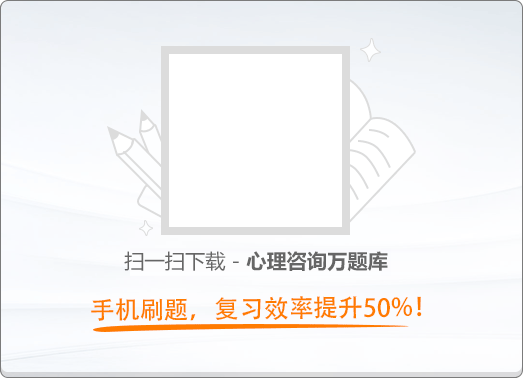90:002014年11月二级心理咨询师《职业道德、理论知识》真题

1
(单项选择题)

• A.

轻伤不下火线，带病坚持工作

• B.

大公无私，不考虑个人利益

• C.

绝不“跳槽”

• D.

集体利益与个人利益兼顾，以集体利益为重

• A
• B
• C
• D

2
(单项选择题)

• A.

企业形象的本质是企业信誉

• B.

企业形象主要体现在厂容厂貌上

• C.

树立企业形象的根本途径是加强媒体宣传

• D.

社会公众的看法总有分歧，对企业形象的认识莫衷一是

• A
• B
• C
• D

3
(单项选择题)

• A.

人道主义

• B.

利己主义

• C.

集体主义

• D.

唯物主义

• A
• B
• C
• D

4
(单项选择题)

• A.

由于地位不同，导致双方在人格上不尽平等

• B.

由于权力不同，导致双方对企业的重视程度不尽相同

• C.

由于工作性质不同，双方在道德规范和道德标准上存在一定差异

• D.

由于追求不同，导致双方在利益目标上不尽统一

• A
• B
• C
• D

5
(单项选择题)

• A.

“不知道”

• B.

“请稍候”

• C.

“你需要点什么”

• D.

“请原谅”

• A
• B
• C
• D

6
(单项选择题)

• A.

态度谦卑

• B.

表情从容

• C.

举止潇洒

• D.

形象出众

• A
• B
• C
• D

7
(单项选择题)

• A.

职业技能比职业道德重要

• B.

提高职业道德有助于提高职业技能

• C.

职业技能是立身之本

• D.

职业道德素质与职业技能素质没有任何关联

• A
• B
• C
• D

8
(单项选择题)

• A.

诚实容易守信难

• B.

诚信是不可检验、证实的

• C.

诚信既指不欺人，也指不自欺

• D.

是否诚信以対方表现为前提

• A
• B
• C
• D

9
(多项选择题)

• A.

整合功能

• B.

休闲功能

• C.

自律功能

• D.

健身功能

• A
• B
• C
• D

10
(多项选择题)

• A.

范围上的有限性

• B.

内容上的稳定性和连续性

• C.

形式上的多样性

• D.

应用效果上的不确定性

• A
• B
• C
• D

11
(多项选择题)

《公民道德建设实施纲要》所规定的职业道德规范有（　）。

• A.

办事公道

• B.

团结互助

• C.

服务群众

• D.

奉献社会

• A
• B
• C
• D

12
(多项选择题)

• A.

做大做强

• B.

平稳、快速

• C.

以人为本

• D.

全面、协调、可持续

• A
• B
• C
• D

13
(多项选择题)

• A.

把生活标准降到最低限

• B.

不该花的钱不花，该节省的资源要节省

• C.

节用有度，合理节制

• D.

节俭不是对所有人的统一要求

• A
• B
• C
• D

14
(多项选择题)

• A.

职业行为规范

• B.

具有一定强制性

• C.

企业领导意志的体现

• D.

检验员工职业素养的重要尺度

• A
• B
• C
• D

15
(多项选择题)

• A.

法律面前一律平等

• B.

享受权利，尽到义务

• C.

只要不犯罪就是手法

• D.

敢于用法维护自身权益

• A
• B
• C
• D

16
(多项选择题)

• A.

互相学习是团结互助的具体体现

• B.

为了实现共同目标一致行动属于团结

• C.

团结互助之中同样存在着合理竞争

• D.

只有朋友之间才能实现真正的团结互助

• A
• B
• C
• D